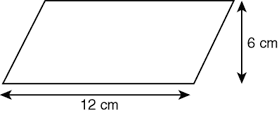Definition: parallelogram from The Penguin Dictionary of Science

A ➤quadrilateral with two pairs of parallel sides.

Summary Article: parallelogram
From The Hutchinson Unabridged Encyclopedia with Atlas and Weather Guide

A quadrilateral (a shape with four sides) with opposite pairs of sides equal in length and parallel, and opposite angles equal. The diagonals of a parallelogram bisect each other. Its area is the product of the length (l) of one side and the perpendicular distance (height h) between this and the opposite side; the formula is A = l × h. In the special case when all four sides are equal in length, the parallelogram is known as a rhombus, and when the internal angles are right angles, it is a rectangle or square.

For example, to find the area of a parallelogram 12 cm long and 6 cm high:A = l × h, so

A = 12 × 6 = 72 cm2

images

parallelogram© RM, 2018. All rights reserved.

### Related Articles

##### Full text Article parallelogram
Philip's Encyclopedia

Quadrilateral (four-sided plane figure) having each pair of opposite sides parallel and equal. Both the opposite angles of a parallelogram are...

##### Full text Article parallelogram
The Penguin English Dictionary

/parəleləgram/ noun a quadrilateral with opposite sides parallel and equal [ via late Latin from Greek parallēlogrammon , neuter of ...

##### Full text Article parallelogram
The Penguin Dictionary of Mathematics

A quadrilateral that has both pairs of opposite sides equal. The area of a parallelogram is the length of any side multiplied by the...

See more from Credo